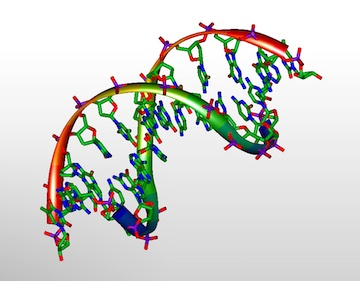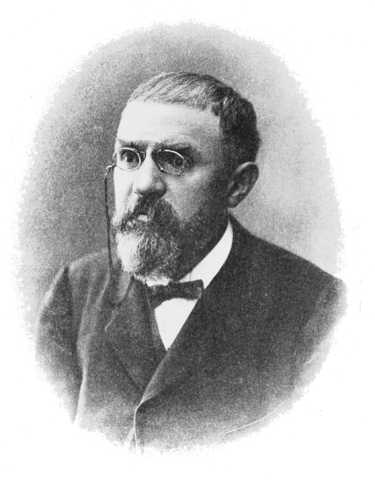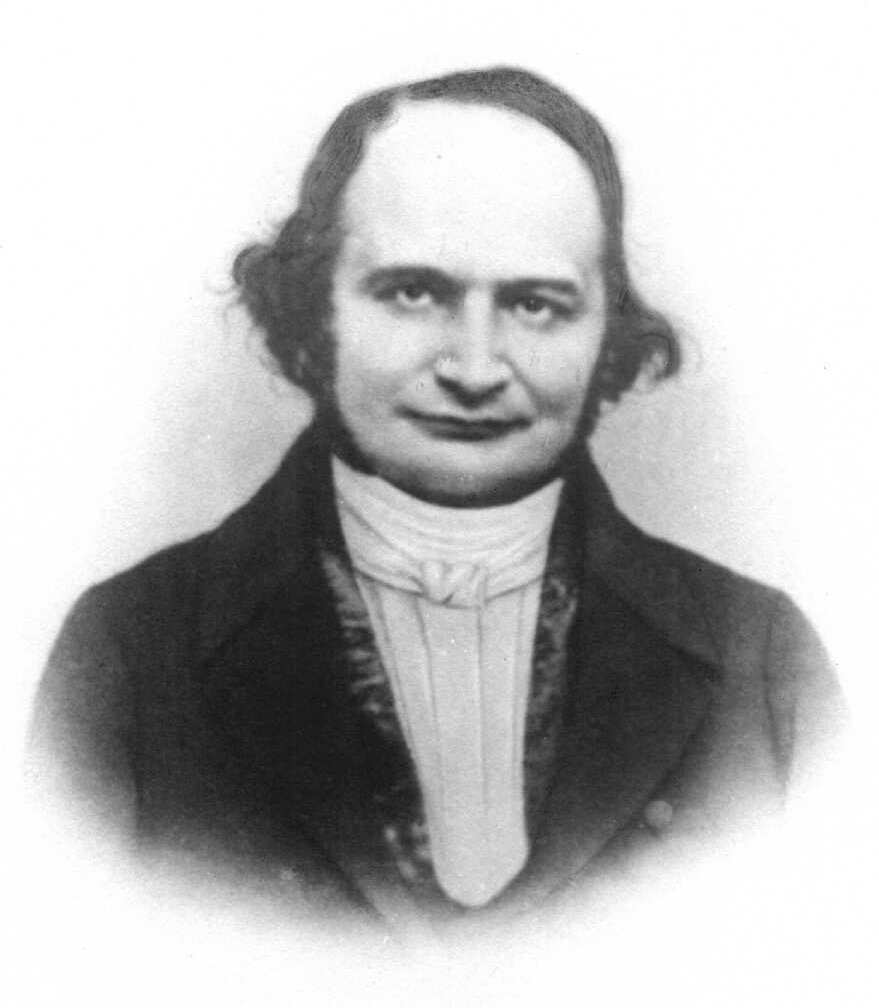Johannes Vermeer: Girl with a pearl earring

# Forum for matematiske perler(og kuriositeter)

Om Forum for matematiske perler (og kuriositeter)
Tidligere år: 2001/2002 · 2002/2003 · 2003/2004 · 2004/2005 · 2005/2006 · 2006/2007 · 2007/2008 · 2008/2009 · 2009/2010 · 2010/2011 · 2011/2012 · 2012/2013 · 2013/2014 · 2014/2015 · 2015/2016 · 2016/2017 · 2017/2018 · 2018/2019 · 2019/2020 · 2020/2021.

## Foredrag 2021–2022

— neste foredrag blir annonsert her i tidens fylde —

### Mats Ehrnström: The Hardy–Weinberg Principle and Evolutionary Genetics🔗Sted:
Lunsjrommet i 13. etg., Sentralbygg 2
Tid:
–13:00
Video / presentasjon
Opptak av foredraget (panopto)
Presentasjon (pdf)

Wandering off from my usual topics, I intend in this talk to explore a very simple though fundamental principle in evolutionary genetics, partly attributed to renowned mathematician G H Hardy. Roughly from the early 2000s and onward, the methods and results of determining the movements of certain genes and groups of genes in time and space has reached the public in increasing mass in findings about ancient ancestry and population groups. We look in this talk at some of the underlying principles for these findings, and especially the Hardy–Weinberg principle.

### Peter Lindqvist: From the solar system to $N$-body problems🔗Sted:
Lunsjrommet i 13. etg., Sentralbygg 2
Tid:
–13:00

The stability of the Solar System was “proved” already by Laplace, Lagrange, Poisson, and by many later scientists. At the end of the 19th century, investigations of Poincaré indicated that no definite answer in any form is possible.

A mathematical (over)simplification is the $N$-body problem consisting of $N$ masspoints obeying the equations $$m_{\iota}\frac{d^{2}\mathbf{r}_{\iota}}{dt^2} =G \sum_{j\neq \iota} m_{\iota} m_j \frac{\mathbf{r}_j-\mathbf{r}_{\iota}} {|\mathbf{r}_j-\mathbf{r}_{\iota}|^3} \qquad (\iota = 1,2,...,N)$$ of motion, ignoring everything else but Newton's Gravitational Law.

The 2-body problem has a well-known solution, but already the 3-body problem offers many strange situations: we shall see that three masspoints with initial velocities zero can escape to infinity, even if there are no collisions (Meissel 1893, Burrau 1913, Szebehely 1967). Among the new results for the 4-body problem is a configuration of four non-colliding masspoints such that one of them reaches the point at infinity in finite time; J. Xue 2020. (This arcane phenomenon is impossible in the 3-body problem.)

I shall start with the 3-body problem and discuss Sundman's explicit solution. –The Levi-Cività transform of coordinates provides an illustration of binary collisions in the plane. It has no counterpart in space, but the Kustaanheimo–Stiefel transform $$x_1+x_2\,\mathbf{i} +x_3\,\mathbf{j}+x_4\,\mathbf{k}\, =\,\bigl(u_1+u_2\mathbf{i}+u_3\mathbf{j}+ u_4 \mathbf{k}\bigr)^2$$ with quaternions is used to regularize the motion near a collision. (A square root is calculated in 4 dimensions; then one variable is killed, say $x_4=0$, to get back to 3 dimensions.)

The corresponding problems in Einstein's general theory of relativity are not well understood.

### Christian Skau: Why is 1093 an interesting prime?🔗Sted:
Lunsjrommet i 13. etg., Sentralbygg 2
Tid:
–13:00
Slides

This all started with a question that Abel posed in Crelle’s journal in 1828, under the heading “Aufgaben und Lehrsätze”. The question was answered by Jacobi and appeared in the same issue of the journal. Astonishingly, a special case of Abel’s question, not addressed by Jacobi, turned out to be intimately related to Fermat’s Last Theorem. Furthermore, the question was also closely related to the Fermat and Mersenne primes, as well as the Bernoulli numbers (and thereby to the Riemann zeta function).